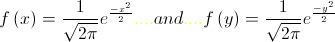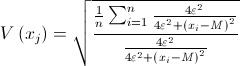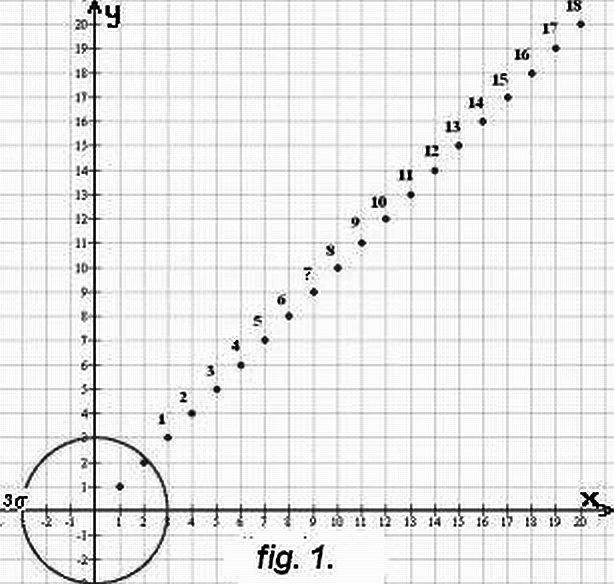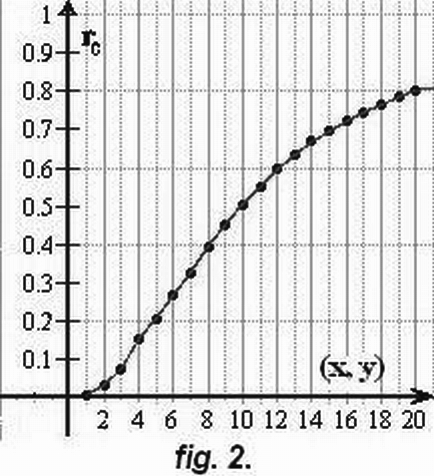Steiner, F., Hajagos, B.:Irresistance of  the classical correlation coefficient

1.Introduction

If the rate of correlation between the data sets xi and yi is to be determined (in both cases i=1, 2, …, n), in the classical mathematical statistics (see e.g. Cramér 1945) the application of the following formula is proposed:(1)

(x and y are the mean values of the data xi  and yi , respectively;  the index c indicates that rc  is a calculated value of the correlation coefficient and not the true rt value of it). For Gaussian distributions in Cramér 1945 also the analytical formula of the probability density function of rc is given for the values of rt and n. This formula is cited as Eq. 2. in Steiner and Hajagos 2008, too. For some cases even the f(rc) curves are shown  in Cramér 1945; a such figure is cited in Steiner 1990 on page 265 for n=10. The curves show really great degree of uncertainty if there are few data-pairs for the calculation of rc , the authors of the present article, however, mention self-critically that in the paragraph ofter Eq. 3. in Steiner and Hajagos 2008 the consequence is too pejoratively ( and too categorically) formulated: the value n=10 is only for our actual theoretical treatments unacceptable small. The formulation, otherwise, in such degree of generality cannot be valid: the expert has in some cases not more data pairs as ten.

2. The concepts of robustness and resistance

Before some decades two new concepts appear in the modern statistics: robustness and resistance (see e.g. Huber 1981).Robust is the statistical method if it is applicable with great enough statistical efficiency for a not too short interval of probability distribution types: the practitioner needs that the method must be applicable for all types which can occur in his discipline. As in the geosciences even the Cauchy-type can occur and Tarantola 1987 proposes the use of such statistical algorithms which are able to handle also the Cauchy-type even if not this distribution is characteristic to the actual problem but it can be justifiably suspected that outliers can occur in a not negligible percent therefore our statistical methods must be applicable also for data which are distributed according to the Cauchy-type.

In the paper Steiner and Hajagos 2008 is shown that Eq.1. cannot be applicated if the xi and yi data are Cauchy-distributed, consequently this formula for the calculation of the correlation coefficient is not robust.

The goal of the present article is, however, to investigate the resistance of Eq.1. , i.e.,the resistance of the classical correlation coefficient. Resistant against outliers is the statistical algorithm if the result is not (or not significantly) influenced by outliers, i.e., by such data  wich are of unknown origin: strange in the sense that they do not belong to the regular data set. Immense causes can originate outliers (trivial examples are the erroneously typed data) and if the resistance is theoretically investigated the artificial outliers are not seldom very far from the regular data set. E.g., Andrews  1972 by investigating the so-called breakdown bounds of different estimates of location used the following 100 data: 100-J data are generated according to the  standard Gaussian probability density functionand the outliers are 100; 200; …; j*100; …J*100. This means that outliers can have values of some thousands, too, although the standard Gaussian data are well known in the (-3;+3) interval („3s  rule”).

On page 184 in the book Steiner (ed) 1997 the figure shows that the most frequent value denoted by M (as  estimate of location) using k=2 tolerates even J=10 such outliers: till this limit the M-value is less than 0.02 (instead of the true value zero). The calculation method of the most frequent value as a statistical algorithm can be called justifiably as resistant.

3. Distortion  of the classical correlation coefficient caused by outliers.

Let be assumed the simplest case: the xi  and yi  data are equally standard Gauss-distributed, i.e., the are generated according to the density distributionrespectively; the xi  and yi  data are uncorrelated  (i.e., the true value of the correlation coefficient equals zero: rt =0).

If  99 (xi , yi) data-pairs are according to the foregoings generated, they define 99 points inside the circle on Fig.1. The distortions of the rc-values (see Eq.1.) caused by one single outlier (only 1% of the data-pairs) were investigared if this outlier is near or far from the circle of the regular points. On Fig.1 the simple „menu” of outliers is given by points with series number; the x=y outliers are the followings: 1:(3;3);  2:(4;4); …; 18:(20;20).

For outlier-free case the f(rc)- curve is given for 100 Gaussian distributed points inside the circle on Fig.1 in Steiner and Hajagos 2008. The half-value width of this curve is greater than 0.2 ; consequently, Monte-Carlo calculations are needed to each outlier to have that distorted rc-value which can be accepted as the most probable one. In the justcited article only ten result was given for only one outlier (which  corresponds to the (10;10) in our actual menu). These values bring about 0.5 – insted of zero –demonstrated that the classical correlation coefficient is not resistant. This justification of the existence of irresistance was enough as the main goal of this article was to present resistant alternatives for the calculation of the correlation coefficient (see Eq.8) and to investigate these new resistant characteristics.

In the present paper the regularity of the distortions caused by the outliers shown in Fig.1 are to be determined accurately enough, therefore for each outlier, 101 times were 99 Gaussian points generated, 101 rc-values were calculated according to Eq.1 and the median of these rc-values were determined; this procedure was 5 times repeated and finally the median of the 5 medians were accepted as a properly accurate result. These rc-values are represented in Fig.2 for each outlier defined in Fig.1. The distortion equals 0.2 even if the outlier is not too far:      ( see e.g. the case of the (5;5) outlier series number 3) which is half so far from the origin as the above mentioned (10;10) outlier (remember that in Steiner and Hajagos 2008 the distorting effect of this was shown in Fig.1).

4. A short not to the meaning of the word „outlier”

The expression „outlier” has pejorative meaning if we have to characterize the whole set of data ( or the whole of pairs of data as in the above discussed cases): we have seen that the result can be even misleading in presence of a single outlier. The outliers are, however, not always „wrong” data (e.g., they were erroneously typed); in the contrary, in som discipline of science they contain the most valuable informations. In the following paper of J. Verő 2008 fortunately there are given also concrete examples of such type. Consequently, there is given every reason to hope that in this way  the reader is correctly informed about the Janus-faced character of the outliers.

A last remark: in some cases the most frequent value M and the dihesion e of the xi data (i=1, 2, …, n ) are able to characterize the value of information of the outlier (denoted by V(xj)) if the letter can be accepted approximately proportional to the distance between the valuable outlier and the dense lying set of points:

nbsp;(2)

The expert of the discipline decides if this way to the selection and valuation of the far lying  data is convenient for him or n

Captions

Fig. 1. On the circle of radius 3s lie the points with 99.7% probability if they represent the
value-pairs of two independent standard Gaussian distributions. Consequently thepoints marked with serial numbers are in this case outliers. ( Near the origin two
„inliers” are also given without series numbers.)

Fig. 2. The most probable rc-values calculated according to the classical formula of thecorrelation coefficient (see Eq. 1.) if 99 value-pairs are generated according to the
standard Gaussian rule ( see the circle on Fig. 1.) and only a single outlying point is
taken into consideration ( characterised by the same value of x and y, see Fig. 1.). The
Fig. 1. in Hajagos and Steiner 2008 corresponds to the case x=y=10 resulting in the rc-
Value of 0.5 but even if the outlier is half so far, i.e., x=y=5, rc=0.2 holds instead of  zero.

# References

Andrews, D. F.,Bickel, P. J., Hampel, F. R., Huber, P. J., Rogers, W. H., Tukey, J. W. 1972:

Robust Estimates of Location. Survey and Advances. Princeton University
Press, Princeton, New Jersey, 373 p.
.Cramér, H. 1945: Mathematical Methods of Statistics.
Almquist & Wiksells, Uppsala, 575 p.
Huber, P. J. 1981: Robust Statistics. Wiley, NewYork, 308 p.
Tarantola, A. 1987: Inverse Problem Theory. Elsevier, Amsterdam, 613 p
Steiner, F. 1990: Introduction to Geostatistics ( in Hungarian).